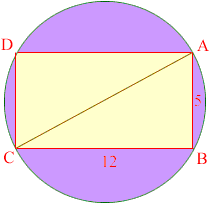Quandaries and Queries Name: Abdu Who is asking: Student Level: Secondary Question: A rectangle ABCD is inscribed in a circle. If the length of AB is 5 and length of BC is 12, what is the area of circle outside the rectanlge ABCD? A 40.8 B 53.1 C 72.7 D 78.5 E 81.7 Hi Abdu, Draw a diagram.The rectagle is 5 units by 12 units so its area is 512 = 60 square units. To find the area of the circle you need its radius. The line CA is a diameter of the circle and triangle ABC is a right triangle so you can use Pythagoras' theorem to find the length of CA. Can you finish the problem now? Penny Go to Math Central